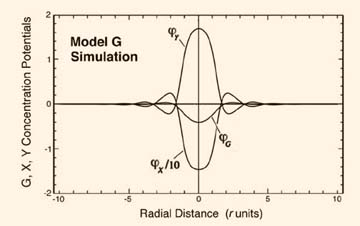# SQK Simulations Question

Paul,
On page 79 of your new edition of SQK, regarding the simulated nucleation of a neutral particle, you state that no net decrease in G below the steady state value is seen. That is not quite apparent to me from the simulation. Although biased more toward neutral than in Figure 17, the area under the G curve still seems to be net negative. I suppose the curve is just narrow at the particle, and hence, the area is essentially zero. Perhaps that is the point? Perhaps the figures are not quite accurate? What am I missing?Answer to your question: This simulation of a neutral particle (shown below) plots concentration vs. radial distance from the particle center.  But since volume increases as the cube of radius, the volume of the adjacent high-G shell will be found to be much greater than that of the low-G core region.  To sum up the total G-on discrepancy above and below the zero potential line we must multiply the concentration value by the volume increment that it resides in.  When we do this, it is found that the G-on excess in the shell approximately equals the G-on deficit in the core.  So both average out to zero.  The same should be found by analyzing successive cycles of the neutral particle’s Turing wave pattern.
Paul LaViolette,  Jan. 28, 2011